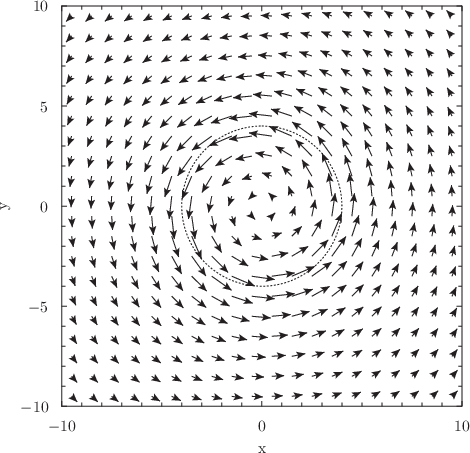## 8.2.6 Arrows

The following plot styles allow arrows or lines to be drawn on graphs with positions dictated by a series of data points:

The plot style of arrows is an alias for arrows_head. Each of these plot styles take four columns of data on two-dimensional plots –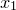,,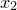and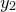– or six columns of data on three-dimensional plots with additional-coordinates. Each data point results in an arrow being drawn from the point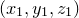to the point. The three plot styles differ in the kinds of arrows that they draw: arrows_head draws an arrow head on each arrow at the point; arrows_nohead draws simple lines without arrow heads on either end; arrows_twohead draws arrow heads on both ends of each arrow.

Example: A diagram of fluid flow around a vortex

In this example we produce a velocity map of fluid circulating in a vortex. For simplicity, we assume that the fluid in the core of the vortex, at radii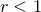, is undergoing solid body rotation with velocity, and that the fluid outside this core is behaving as a free vortex with velocity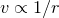. First of all, we use a simple python script to generate a data file with the four columns:
from math import *
for i in range(-19,20,2):
for j in range(-19,20,2):
x = float(i)/2
y = float(j)/2
r = sqrt(x**2 + y**2) / 4
theta = atan2(y,x)
if (r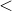1.0): v = 1.3*r
else : v = 1.3/r
vy = v * cos(theta)
vx = v * -sin(theta)
print "%7.3f %7.3f %7.3f %7.3f"%(x,y,vx,vy)
This data can then be plotted using the following Pyxplot script:
set size square
set width 9
set nokey
set xlabel ’x’
set ylabel ’y’
set trange [0:2*pi]
plot’vortex.dat’ u 1:2:(\$1+\$3):(\$2+\$4) w arrows,parametric 4*sin(t):4*cos(t) w lt 2 col black